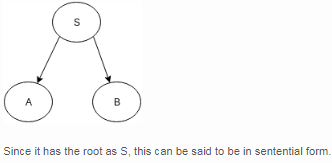Courses

# Test: DPDA & Context Free Languages

## 10 Questions MCQ Test Theory of Computation | Test: DPDA & Context Free Languages

Description
This mock test of Test: DPDA & Context Free Languages for Computer Science Engineering (CSE) helps you for every Computer Science Engineering (CSE) entrance exam. This contains 10 Multiple Choice Questions for Computer Science Engineering (CSE) Test: DPDA & Context Free Languages (mcq) to study with solutions a complete question bank. The solved questions answers in this Test: DPDA & Context Free Languages quiz give you a good mix of easy questions and tough questions. Computer Science Engineering (CSE) students definitely take this Test: DPDA & Context Free Languages exercise for a better result in the exam. You can find other Test: DPDA & Context Free Languages extra questions, long questions & short questions for Computer Science Engineering (CSE) on EduRev as well by searching above.
QUESTION: 1

### Context free grammar is called Type 2 grammar because of ______________ hierarchy.

Solution:

Chomsky hierarchy decide four type of language :Type 3- Regular Language, Type 2-Context free language, Type 1-Context Sensitive Language, Type 0- Unrestricted or Recursively Ennumerable language.

QUESTION: 2

### a→bRestriction: Length of b must be atleast as much length of a.Which of the following is correct for the given assertion?

Solution:

A context-sensitive grammar (CSG) is a formal grammar in which the left-hand sides and right-hand sides of any production rules may be surrounded by a context of terminal and non terminal symbols. Context-sensitive grammars are more general than context-free grammars, in the sense that there are some languages that cannot be described by context-free grammars, but can be described by CSG.

QUESTION: 3

### From the definition of context free grammars, G=(V, T, P, S) What is the solution of VÇT?

Solution:

V is the set of non terminal symbols while T is the st of terminal symbols, their intersection would always be null.

QUESTION: 4

If P is the production, for the given statement, state true or false.P: V->(V∑T)* represents that the left hand side production rule has no right or left context.

Solution:

Here the production P is from the definition of Context free grammar and thus, has no right or left context.

QUESTION: 5

There exists a Context free grammar such that:X->aXWhich among the following is correct with respect to the given assertion?

Solution:

The grammar with right recursive production is known as Right recursive grammar. Right recursive production is of the form X->aX where a is a terminal and X is a non terminal.

QUESTION: 6

If the partial derivation tree contains the root as the starting variable, the form is known as:

Solution:

Example: For any grammar, productions be:
S->AB
A->aaA| ^
B->Bb| ^
The partial derivation tree can be drawn as:QUESTION: 7

Find a regular expression for a grammar which generates a language which states :
L contains a set of strings starting wth an a and ending with a b, with something in the middle.

Solution:

The grammar for the same language can be stated as :
(1) S → aMb
(2) M → A
(3) M → B
(4) A → e
(5) A → aA
(6) B → e
(7) B → bB

QUESTION: 8

Which of the following is the correct representation of grammar for the given regular expression?
a(aUb)*b

Solution:

The basic idea of grammar formalisms is to capture the structure of string by
a) using special symbols to stand for substrings of a particular structure
b) using rules to specify how the substrings are combined to form new substrings.

QUESTION: 9

A CFG consist of the following elements:

Solution:

A CFG consists of:
a) a set of terminals, which are characters of alphabets that appear in the string generated by the grammar.
b) a set of non terminals, which are placeholders for patterns of terminal symbols that can be generated by the nonterminal symbols.
c) a set of productions, which are set of rules to transit from one state to other forming up the string
d) a start symbol, a special non terminal symbol that appears in the initial string generated in the grammar.

QUESTION: 10

A CFG for a program describing strings of letters with the word “main” somewhere in the string:

Solution:

None.